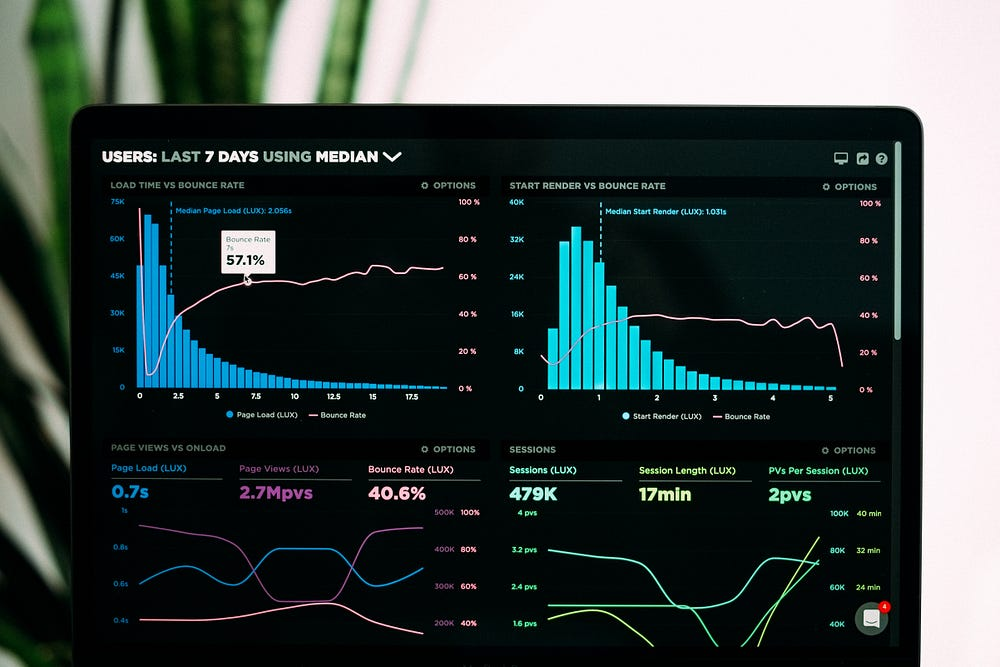# Data Types used in Machine Learning

Source: Deep Learning on Medium# Data Types used in Machine Learning

Numerical

It represent some sort of quantitative measurement.

for example: Height of a people, stock prices

Discrete Data

Integer based, often counts of something

for example: How many times did I fillip “Heads”?

Continuous Data

Has an infinite no of possible values

for example : How much rain fall on a given day?

Categorical Data

Qualitative Data

For example: Gender, Yes/No, Race etc

you can assign some number to categorical data but they don’t have any mathematical meaning

Ordinal Data

Mixture of numerical and categorical

categorical data has mathematical meaning

For example: Movie rating on a scale of 1–5

Rating must be 1,2,3,4,5

They have mathematical meaning. Like rating 1 movie is worse than rating 2 movie